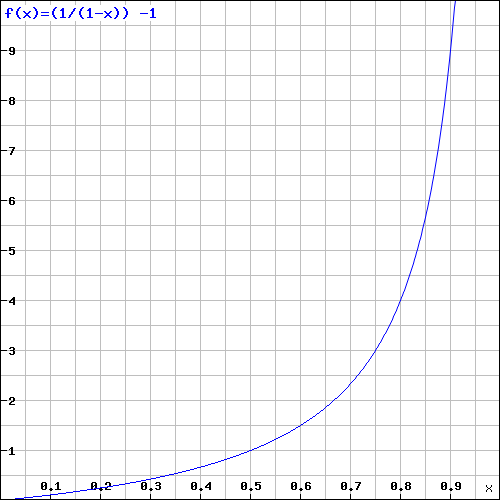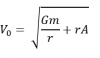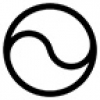## Hypersphere CosmologyAlternative to big-bang

Monday, 01 September 2014 06:31

## Hypersphere RedshiftThe alternative mechanism for redshift works as follows:

Redshift = Z =- 1

Where= observed wavelength,= expected wavelength, and the -1 simply starts the scale at zero rather than 1.

Now wavelength,, time frequency, f, still always equals lightspeed, c .However< c= c -Where d = astronomical distance, A = Anderson acceleration. The Anderson acceleration (the small positive curvature of the hypersphere of the universe) works against the passage of light over the astronomical distance, d. It cannot actually decrease lightspeed but it acts on the frequency component.

So substituting=We obtainTherefore Redshift, Z =- 1

Thus Z becomes just a function of d, astronomical distance, not recession velocity.

To put it in simple terms, redshift does not arise from a huge and inexplicable expansion of the underlying spacetime increasing the wavelength; it arises from the resistance of the small positive gravitational curvature of hyperspherical spacetime to the passage of light decreasing its frequency.

Thus the ‘Hubble Constant’ has a value of precisely zero kilometres per second per magaparsec, but the ‘Hubble Time’ does give a reasonably accurate indication of the temporal horizon and hence the spatial horizon/antipode distance of the hypersphere of the universe.

Redshift and Distance.

We can rearrange the following equation derived from considerations of the effect of a positive spacetime curvature on the frequency of light from distant galaxies: -To give an expression for cosmological distance in terms of redshift; -This simplifies to: -Graphically we can represent this as: -The vertical scale represents Redshift.

The horizontal scale represents observer to antipode distance L, in both space and time in the Hypersphere Cosmology model. In the conventional big bang model it would represent only the time since the emission of the light.

Note that when Z = 0, d = 0.  When Z = 1, d =When Z = 10, d ~ 0.91 L

And when Z →∞, d = L

A consideration of Frequency Shift gives a more clear and intuitive picture of the effect of the positive spacetime curvature on light from cosmological sources: -

# fo / fe = c - (dA)1/2 / c

• #### HyperSphere Cosmology +Hypersphere Cosmology in a Nutshell   1a) The Universe consists of a Hypersphere (a 3-Sphere or 4-ball) where: -       also
• #### Hypersphere Redshift +The alternative mechanism for redshift works as follows: Redshift = Z =   - 1 Where = observed wavelength, = expected wavelength, and the -1
• #### Hypersphere Rotation +The Gödel 3d rotating universe has this solution derived from General Relativity: - “Matter everywhere rotates relative to the compass of
• #### Hypersphere Holometry - A Prediction +As a consequence of the hypothesis that the universe exists as a hypersphere where   the main parameters of the
• #### VHC. Vorticitating Hypersphere Cosmology +The overwhelming majority of university and state funded cosmologists believe that the observable universe has expanded from a much smaller
• #### Hypersphere Visualisation & Lensing +This paper provides a method of visualising the four dimensional hypersphere as a perspective construction in three dimensions, and it
• #### Sacred Geometry +

As faith began to make way for reason during the Enlightenment, humans began to deduce the relationships between certain mathematical
• #### Starships +

Houston we have a problem. If the human race does not develop starships that can reach other star systems as
• #### Quantum & Probability +

I have repeatedly asserted in many of my magical and scientific books and papers that this universe runs on probability
• #### Ur Chaos Theory +

In this paper 'Chaos' denotes randomness and indeterminacy, not the mere so-called 'deterministic chaos' of systems with extreme sensitivity to
• 1